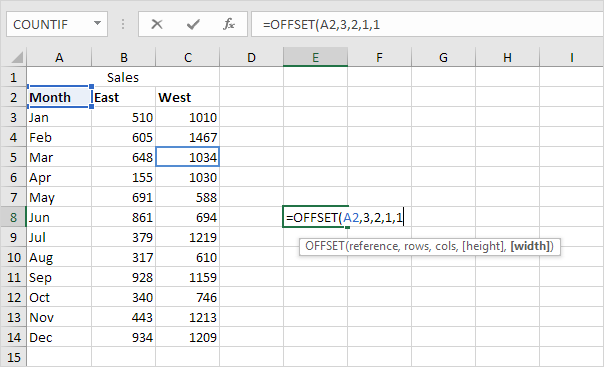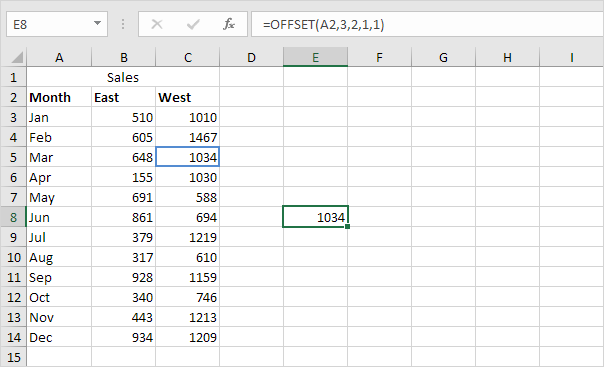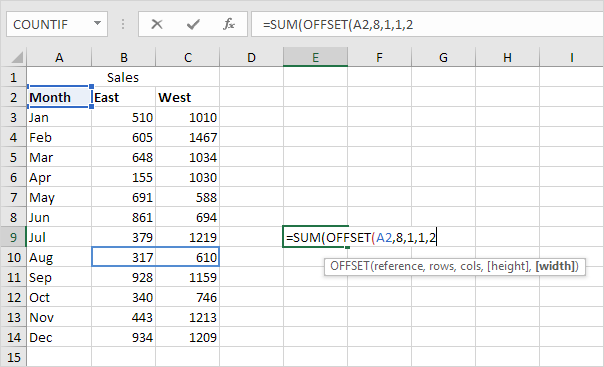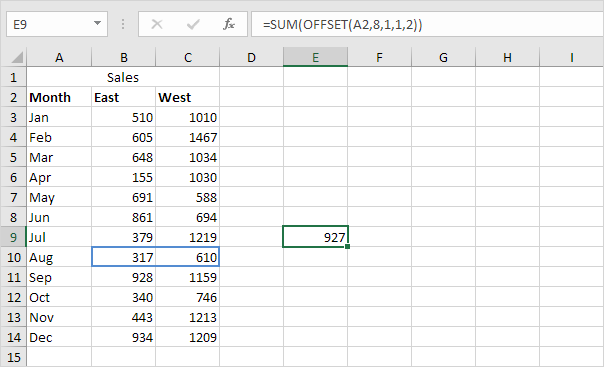## Excel Office

Excel How Tos, Tutorials, Tips & Tricks, Shortcuts

# Offset in Excel

The OFFSET function in Excel returns a cell or range of cells that is a specified number of rows and columns from a cell or range of cells.

OFFSET function Syntax;

`OFFSET(reference, rows, cols, [height], [width])`

1. The OFFSET function below returns the cell that is 3 rows below and 2 columns to the right of cell A2. The OFFSET function returns a cell because the height and width are both set to 1.

Also See:   How to create dynamic named range with OFFSET in ExcelResult:2. The OFFSET function below returns the 1 x 2 range that is 8 rows below and 1 column to the right of cell A2. The SUM function calculates the sum of this range.Result:Note: to return a range (without calculating the sum), select a range of the same size before you insert the OFFSET function. If you want to return a cell or range of cells that is a specified number of rows above or columns to the left, enter a negative number.

Also See:   Average last 5 values in columns in Excel